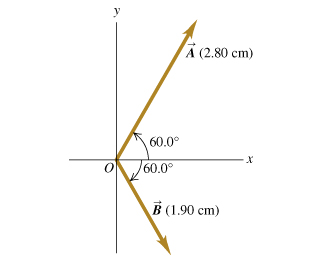# Problem: For the two vectors in the figure , find the magnitude of the vector product vec{A} imes vec{B}.Find the direction of the vector product vec{A} imes vec{B}.Find the magnitude of vec{B} imes vec{A}.Find the direction of vec{B} imes vec{A}.

⚠️Our tutors found the solution shown to be helpful for the problem you're searching for. We don't have the exact solution yet.

###### Problem Details

For the two vectors in the figure, find the magnitude of the vector product .

Find the direction of the vector product .

Find the magnitude of .

Find the direction of .